# Chance-Constrained Model for RCPSP with Uncertain Durations

## Abstract

Project scheduling with uncertain durations becomes a research focus with the development of uncertainty theory. Most researches concerned aim at minimizing project makespan or project cost according to different decision criterions. However, few works considered resource constraint, which initially appeared in deterministic resource-constrained project scheduling problem (RCPSP) and reflects the reality of a project. In this paper, RCPSP with uncertain activity durations, or uncertain RCPSP (URCPSP), is explored. Our aim is to minimize project makespan with certain belief degree. A corresponding uncertain model is built based on chance-constrained programming. To solve the model, a hybrid intelligent algorithm integrating genetic algorithm and an uncertain serial schedule generation scheme is designed and tested in some numerical examples. This work may provide some advices for the risk-averse project manager.

## Introduction

Project scheduling is to assign activity starting times according to scheduling objectives, such as minimal project makespan and minimal project cost . The problem can be divided into many subproblems, including resource-constrained project scheduling problem (RCPSP), resource leveling problem (RLP), and time-cost trade-off problem (TCTP). As a basic project scheduling problem, RCPSP has been a research focus since Pritsker et al.  proposed a mathematical model. The problem aims at minimization of the makespan with consideration of precedence and resource constraints . Researches in the early phase were done with the assumptions of complete information and deterministic environment. For a given project, a baseline schedule, a list of activity starting times, can be obtained by solving deterministic RCPSP. However, the baseline schedule is vulnerable when being executed in an indeterminate environment. In reality, there are considerable indeterminacies (accident, resource breakdown, unreliable deliveries, etc.), which may result in an infeasible baseline schedule. Hence, it is necessary to consider indeterminate factors when solving a project scheduling problem.

In Herroelen and Leus , five directions were distinguished for project scheduling in an indeterminate environment: reactive scheduling, stochastic project scheduling, fuzzy project scheduling, robust project scheduling, and sensitivity analysis. The stochastic resource-constrained project scheduling problem (SRCPSP), the main context of stochastic project scheduling, is characterized by random activity durations and scheduling policy. Activity durations are assumed to be random variables, and a scheduling policy is used to decide which activities to be started at decision points (the starting time of the project and the finishing times of activities) . Generally, the SRCPSP aims at minimizing the expected makespan by making a limited set of decisions during project execution . For more details about SRCPSP, readers may refer to .

In SRCPSP, activity durations are represented by random variables. The assumption is reasonable when there is enough historical data to precisely estimate variables’ probability distributions. However, it is difficult to get enough historical data for activities seldom or never executed. This situation is common with the consideration of the uniqueness of the project. Therefore, new theories instead of probability theory are needed to solve problems with such variables . One optional theory is uncertainty theory, initiated by Liu  and refined by Liu . For now, the new theory has been successfully applied to the following fields: option pricing problem , variation analysis , shortest path problem , facility location problem , uncertain process , stock problem [22, 23], inventory problem [24, 25], assignment problem , newboy problem , production control problem [28, 29], supply chain pricing problem , and uncertain random process . In the field of project scheduling, some important researches were also done. Zhang and Chen  proposed an expected makespan minimization model for project scheduling problem with uncertain durations and total cost chance constraint. Ding and Zhu  established a multi-objective pessimistic value model and time range measure optimization model for uncertain project scheduling based on different management requirements. Ji and Yao  explored a project scheduling problem where both the duration times and the resource allocation times are uncertain variables. A multi-objective model was obtained to minimize the total cost and the overtime. Ke et al.  researched project scheduling problem in the environment with uncertainty and randomness. An uncertain random expected cost minimization model was built and solved by a hybrid intelligent algorithm.

As far as we know, few researches pay attention to project scheduling problem with uncertain activity durations as well as resource constraint. In fact, resource constraint is common as resources in project execution are always limited. Additionally, most literature concerning uncertain project scheduling aims at minimizing the expected makespan and ignores the reliability of the optimal expected makespan. Therefore, the project scheduling problem in this paper possesses three characteristics simultaneously: uncertain activity durations, resource constraints, and belief degree for the objective value. In detail, an uncertain model based on chance-constrained programming instead of expected value model is proposed to minimize project makespan with some belief degree, which is applicable to the risk-averse decision-maker who wants to realize the project schedule with a pretty high belief degree.

The reminder of this paper is as follows: the “Preliminaries” section introduces some basic concepts in uncertainty theory. The “Formulations and Models” section describes RCPSP with uncertain activity durations, or uncertain RCPSP (URCPSP), in detail and proposes a corresponding chance-constrained model to satisfy the demands of risk averter. Furthermore, we transform the proposed model into a crisp programming model. To solve the model, a genetic algorithm is designed in the “GA-based Algorithms” section. The “Numerical Examples” section conducts some numerical examples. Finally, conclusions are drawn in the “Conclusion” section.

## Preliminaries

As a branch of axiomatic mathematics, uncertainty theory has been well developed in many real problems. In this section, some basic concepts and theorems in uncertainty theory are introduced.

Let Γ be a nonempty set,a σ-algebra over Γ, and each element Λ inis called an event. Uncertain measure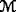, initiated by Liu  and refined by Liu , is a function fromto [0,1]. It is defined over the following four axioms.

### Axiom1.

(Normality axiom)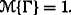.

### Axiom2.

(Duality axiom)for any event Λ.

### Axiom3.

(Subadditivity axiom) For every countable sequence of events {Λ i }, i= 1,2,, we have: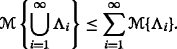### Axiom4.

(Product measure axiom) Letbe uncertainty spaces for k=1,2, The product uncertain measureis an uncertain measure satisfying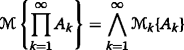where A k are arbitrarily chosen events from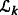for k=1,2,, respectively.

### Definition1.

 An uncertain variable is a measurable function ξ from an uncertainty spaceto the set of real numbers, i.e., for any Borel set B of real numbers, the set

\begin{aligned} &\{\xi\in B\}=\{\gamma\in \Gamma \ \big|\ \xi(\gamma)\in B\} \end{aligned}

is an event.

The uncertainty distribution is indispensable to establish practical uncertain optimization models.

### Definition2.

 The uncertainty distribution Φ of an uncertain variable ξ is defined byfor any real number x.

An uncertainty distribution Φ is confirmed to be regular if its inverse function Φ −1(α) exists uniquely for each α[0,1].

### Definition3.

 Let ξ be an uncertain variable. The expected value of ξ is defined byprovided that at least one of the above two integrals is finite.

### Lemma1.

 Let ξ be an uncertain variable with uncertainty distribution Φ. If the expected value exists, then

$$E[\xi]=\int_{0}^{+\infty} (1-\Phi(x))\textup{d} x-\int_{-\infty}^{0} \Phi(x)\textup{d} x.$$

### Lemma2.

 Let ξ be an uncertain variable with regular uncertainty distribution Φ. If the expected value exists, then

$$E[\xi]={\int_{0}^{1}} \Phi^{-1}(\alpha)\textup{d} \alpha.$$

### Lemma3.

 Let ξ 1,ξ 2,,ξ n be independent uncertain variables with regular uncertainty distributions Φ 1,Φ 2,,Φ n , respectively. A function f(x 1,x 2,,x n ) is strictly increasing with respect to x 1,x 2,,x m and strictly decreasing with respect to x m+1,x m+2,,x n . Then ξ=f(ξ 1,ξ 2,,ξ n ) is an uncertain variable with inverse uncertainty distribution

\begin{aligned} \Psi^{-1}(\alpha)= f(\Phi_{1}^{-1}(\alpha),\cdots,\Phi_{m}^{-1}(\alpha),\Phi_{m+1}^{-1}(1-\alpha),\cdots,\Phi_{n}^{-1}(1-\alpha)). \end{aligned}

## Formulations and Models

### Problem Description

A project containing n activities can be described by an activity-on-the-node network G(N,A) as shown in Fig. 1. The set of nodes N={1,2,,n} represents activities, and the set of arcs A denotes finish-start, zero-lag precedence relations between activities. Activities 1 and n are dummy activities as they do not consume time and resource and only signify the project starting point and finishing point, respectively. Specially, durations of all activities in URCPSP are represented by an uncertain vector $${\boldsymbol {d}} =\{0,\widetilde {d_{2}},\cdots, \widetilde {d}_{n-1},0\}.$$ Moreover, there are totally K types of renewable resources, and each of them has a constant availability a k , k=1,2,,K.

The URCPSP aims at minimizing the project makespan with uncertain activity durations. Solving URCPSP is a dynamic decision process. The manager decides to start which feasible activity at each decision point, including project starting time and activity finishing times. In the decision process, the manager can only utilize partial information which appears before his decision point. This process will be simulated in our algorithm.

### Chance-Constrained Model

The URCPSP aims at minimizing the project makespan, namely s n , the starting time of activity n. Most literature concerned aimed at minimizing the expected makespan and ignored the reliability of the optimal expected makespan . Actually, the optimal value of the expected model can be realized with a belief degree of about 0.5 in most cases. In other words, the realized makespan is larger than the expected one with a pretty high belief degree. This is unacceptable for a risk averter. Moreover, the risk preference of a decision maker may also vary in an uncertain environment. To satisfy the demand of the risk-averse decision maker, an uncertain model based on the philosophy of chance-constrained programming  is proposed as follows: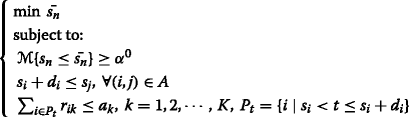where α 0 is a confidence level.

In the above model, the objective function is to minimize the makespan with belief degree α 0, enforced in the first constraint. The second constraint ensures the earliest starting time of activity j to be larger than the finishing time of its predecessor activity i. The third constraint guarantees that the resource demand for each resource type is always below its availability. Specifically, P t represents the set of underway activities at time t, r ik is the demand of activity i for resource k, and a k is the availability of resource k.

Activity durations are uncertain variables. As a result, the finishing time of each activity is changeable. With a given activity list AL, an executing order of activities, $$\tilde {f_{i}}$$, the finishing time of activity i, can be calculated as follows: $$\tilde {f_{i}}(AL)=s_{i}+\tilde {d}_{i}.$$

Without resource constraint, the starting time of activity i can be computed considering precedence relation as follows:

$$s_{i}(AL)=\max\limits_{(j,i)\in A} \tilde{f_{j}}(AL).$$

However, the formula cannot hold with resource constraint. Some activity is feasible in precedence relation logic if all of its predecessors have been finished, while it may be infeasible for lacking available resource. In other words, an activity has predecessors in a precedence relation logic as well as in resource logic. To produce a feasible schedule for a resource-constrained project scheduling problem effectively, a resource flow network was presented by Artigues and Roubellatp . If there is a resource flow, an extra relation is added into the original network between activities without precedence relation. Thus, an extended precedence relation set A is developed. Combined with the side constraint proposed by Ma et al. , the starting time of activity i can be calculated by

$$s_{i}(AL)=\max\limits_{AL^{m}<AL^{i}} s_{m}(AL) \vee \max\limits_{(j,i)\in A^{*}} \tilde{f_{j}}(AL).$$

where A L i is the position of activity i in activity list AL.

Provided that the duration of activity i has a regular distribution Φ i (x) and an inverse uncertainty distribution $$\Phi ^{-1}_{i}(\alpha)$$, α(0,1], and s i (A L) has an inverse uncertainty distribution $$\Psi _{i}^{-1}(AL,\alpha)$$, α(0,1], we can get

$$\Psi_{n}^{-1}(AL,\alpha)= \max\limits_{AL^{m}<AL^{n}} \Psi_{m}^{-1}(AL,\alpha) \vee \max\limits_{(j,n)\in A^{*}} \Big(\Psi_{j}^{-1}(AL,\alpha)+\Phi_{j}^{-1}(\alpha) \Big).$$

Accordingly, the uncertain model can be transformed as follows:

\begin{aligned} \left\{ \begin{array}{ll} \min \Psi_{n}^{-1}(AL,\alpha^{0}) \\ \ \text{subject to:}\\ \ \ s_{i}+ d_{i}\le s_{j},\ \forall (i,j)\in A\\ \ \ \sum_{i\in P_{t}}r_{ik}\le a_{k},\ k=1,2,\cdots,K, \ \ P_{t}=\{i\mid s_{i}\le t < s_{i}+d_{i}\}. \end{array} \right. \end{aligned}

## GA-based Algorithms

Deterministic RCPSP is an NP-hard problem. Actually, the exact branch-and-bound algorithm cannot solve no less than 15, 65, and 96.5 % of instances with 20, 30, and 60 activities, respectively . Therefore, URCPSP, an extension of RCPSP, needs to be solved by heuristic or meta-heuristic algorithm. In this section, a hybrid intelligent heuristic algorithm is designed by integrating an uncertain serial SGS (US-SGS), proposed by Ma et al. , with Genetic Algorithm (GA).

### Uncertain Serial SGS for α-Value

As discussed by Kolish and Hartmann , there are several types of feasible solution representations for project scheduling. For URCPSP in this paper, we choose the solution representation activity list AL, which represents the executing order of activities. As solving URCPSP is a dynamic process, the durations of unexecuted activities are unknown. Only after the entire process, all the activity durations can be obtained. The schedule generation scheme (SGS) for a deterministic project problem may become infeasible for uncertain cases. A former work by Ma et al.  designed an US-SGS for uncertain RCPSP. Compared with the serial SGS, a side constraint is added as follows:

$$s_{i}(AL)\le \max\limits_{AL^{m}<AL^{i}} \Big(s_{m}(AL) \Big)$$

where A L i is the position of activity i in AL. The US-SGS can be described as follows: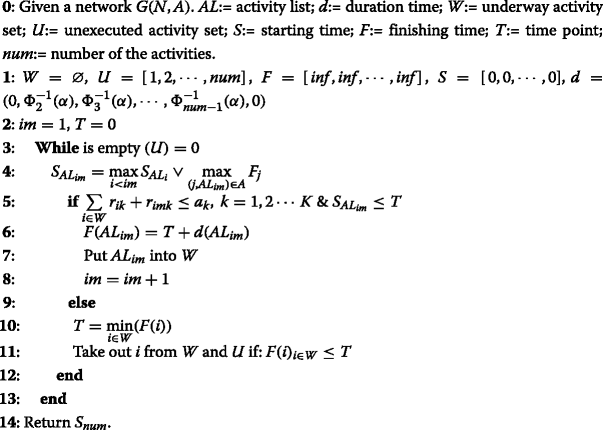### Hybrid Intelligent Algorithm

The US-SGS is embedded into GA in this subsection. The outline of the hybrid intelligent algorithm can be described as follows: first, pop-size solutions are generated randomly as the initial population. Each solution is an activity list, where one activity can only be assigned if all of its precedences have been finished. Second, crossover is performed on the population. Single-point crossover is adopted, and the point is randomly generated. The left part of a child comes from one parent and the right part consists of those remaining activities from another parent by removing activities contained in the left part. In this way, precedence relations are still satisfied. Then mutation is operated to keep the population diversity. A position in solution is chosen randomly as the mutation point. Mutation is realized by changing activity in this position with another activity if precedence relations are still satisfied after the change. Next, the US-SGS is utilized to evaluate each solution. The objective value of each solution is the realized makespan. Then wheel selection is employed to choose the population of the next generation according to objective values. After a certain number of generations, the solution with the best fitness value is reported as the quasi-optimal solution.

## Numerical Examples

In this section, a project with 32 activities and four renewable resources is taken as an example. Some specific information about the project is shown in Table 1, including activity durations, resource requirements, and successors. All the activity durations are assumed to be uncertain variables and described by uncertainty distributions estimated by experts.

If the manager wants to realize the objective value with confidence level 0.9, the chance-constrained model can be rewritten as follows: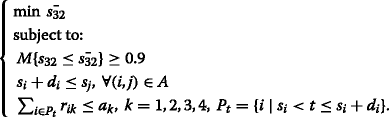The hybrid intelligent algorithm runs ten times with 1000 generations for belief degrees 0.8,0.85,0.9,0.95, and 1, respectively. The quasi-optimal solutions, α 0-values, are provided in Table 2. The result may help a project manager from the following two aspects: first, the project manager can set the project deadline according to a specific request (belief degree); second, a given belief degree corresponds with an optimal schedule.

## Conclusion

In reality, the environment for project execution is full of uncertainties. Considering the uniqueness of a project, it is common that activities are seldom or have never been executed before. As a result, it is difficult to describe activity durations by probability distributions for the lack of historical data. This paper considered a project scheduling problem with uncertain durations and resource constraints. To satisfy the demand of the risk-averse decision maker, an uncertain model was built based on chance-constrained programming. We utilized a special SGS for our problem called US-SGS and added it into the genetic algorithm. Furthermore, some numerical examples were solved with our model and algorithm. We hope our work may provide some references for the risk-averse decision maker.

## References

1. Herroelen, W, Demeulemeester, E, De Reyck, B: A classification scheme for project scheduling. In: J, W (ed.)Project scheduling: recent models, algorithms and applications, pp. 1–26. Kluwer, Amsterdam (1999).

2. Pritsker, AAB, Waiters, LJ, Wolfe, PM: Multiproject scheduling with limited resources: a zero-one programming approach. Manag. Sci. 16(1), 93–108 (1969).

3. Herroelen, W, De Reyck, B, Demeulemeester, E: Resource-constrained project scheduling: a survey of recent developments. Comput. Oper. Res. 25(4), 279–302 (1998).

4. Herroelen, W, Leus, R: Project scheduling under uncertainty: survey and research potentials. Eur. J. Oper. Res. 165(2), 289–306 (2005).

5. Igelmund, G, Radermacher, FJ: Preselective strategies for the optimization of stochastic project networks under resource constraints. Networks. 13(1), 1–28 (1983).

6. Stork, F: Stochastic resource-constrained project scheduling. PhD thesis, Technische Universität Berlin (2001).

7. Möhring, RH, Radermacher, FJ, Weiss, G: Stochastic scheduling problems I: general strategies. ZOR–Zeitschrift für Oper. Res. 28(7), 193–260 (1984).

8. Möhring, RH, Radermacher, FJ, Weiss, G: Stochastic scheduling problems II: set strategies. ZOR–Zeitschrift für Oper. Res. 29(3), 65–104 (1985).

9. Tsai, YW, Gemmill, DD: Using tabu search to schedule activities of stochastic resource-constrained projects. Eur. J. Oper. Res. 111(1), 129–141 (1998).

10. Stork, F: Branch-and-bound algorithms for stochastic resource-constrained project scheduling. Technical Report No. 702/2000. Technische Universität Berlin (2000).

11. Ballestín, F: When it is worthwhile to work with the stochastic RCPSP?J. Sched. 10(3), 153–166 (2007).

12. Ballestin, F, Leus, R: Resource-constrained project scheduling for timely project completion with stochastic activity durations. Prod. Oper. Manag. 18(4), 459–474 (2009).

13. Ashtiani, B, Leus, R, Aryanezhad, M-B: New competitive results for the stochastic resource-constrained project scheduling problem: exploring the benefits of pre-processing. J. Sched. 14(2), 157–171 (2011).

14. Liu, B: Uncertainty theory. 5th edn. Springer, Berlin (2015).

15. Liu, B: Uncertainty theory. 2nd edn. Springer, Berlin (2007).

16. Liu, B: Uncertainty theory. 4th edn. Springer, Berlin (2010).

17. Chen, X: American option pricing formula for uncertain financial market. Int. J. Oper. Res. 8(2), 32–37 (2011).

18. Chen, X: Variation analysis of uncertain stationary independent increment processes. Eur. J. Oper. Res. 222(2), 312–316 (2012).

19. Gao, Y: Shortest path problem with uncertain arc lengths. Comput. Math. Appl. 62(6), 2591–2600 (2011).

20. Gao, Y: Uncertain models for single facility location problems on networks. Appl. Math. Modell. 36(6), 2592–2599 (2012).

21. Liu, B: Extreme value theorems of uncertain process with application to insurance risk model. Soft Comput. 17(4), 549–556 (2013).

22. Peng, J, Yao, K: A new option pricing model for stocks in uncertainty markets. Int. J. Oper. Res. 8(2), 18–26 (2011).

23. Bhattacharyya, R, Chatterjee, A, Kar, S: Uncertainty theory based multiple objective mean-entropy-skewness stock portfolio selection model with transaction costs. J. Uncertainty Anal. Appl. 1(1), 16 (2013).

24. Rong, L: Two new uncertainty programming models of inventory with uncertain costs. J. Inf. Comput. Sci. 8(2), 280–288 (2011).

25. Qin, Z, Kar, S: Single-period inventory problem under uncertain environment. Appl. Math. Comput. 219(18), 9630–9638 (2013).

26. Zhang, B, Peng, J: Uncertain programming model for uncertain optimal assignment problem. Appl. Math. Modell. 37(9), 6458–6468 (2013).

27. Ding, S: Uncertain multi-product newsboy problem with chance constraint. Appl. Math. Comput. 223(3), 139–146 (2013).

28. Ke, H: Uncertain random time-cost trade-off problem. J. Uncertainty Anal. Appl. 2(1), 23 (2014).

29. Liu, B, Yao, K: Uncertain multilevel programming: algorithm and applications. Comput. Ind. Eng. (2014). doi:10.1016/j.cie.2014.09.029.

30. Huang, H, Ke, H: Pricing decision problem for substitutable products based on uncertainty theory. J. Intell. Manuf. (2014). doi:10.1007/s10845-014-0991-7.

31. Gao, J, Yao, K: Some concepts and theorems of uncertain random process. Int. J. Intell. Syst. 30(1), 52–65 (2015).

32. Zhang, X, Chen, X: A new uncertain programming model for project scheduling problem. Inf.: Int. Interdiscip. J. 15(10), 3901–3910 (2012).

33. Ding, C, Zhu, Y: Two empirical uncertain models for project scheduling problem. J. Oper. Res. Soc. (2014). doi:10.1057/jors.2014.115.

34. Ji, X, Yao, K: Uncertain project scheduling problem with resource constraints. J. Intell. Manuf. (2014). doi:10.1007/s10845-014-0980-x.

35. Ke, H, Liu, H, Tian, G: An uncertain random programming model for project scheduling problem. Int. J. Intell. Syst. 30(1), 66–79 (2015).

36. Bruni, ME, Beraldi, P, Guerriero, F, Pinto, E: A heuristic approach for resource constrained project scheduling with uncertain activity durations. Comput. Oper. Res. 38(9), 1305–1318 (2011).

37. Charnes, A, Cooper, WW: Chance-constrained programming. Manag. Sci. 6(1), 73–79 (1959).

38. Artigues, C, Roubellat, F: A polynomial activity insertion algorithm in a multi-resource schedule with cumulative constraints and multiple modes. Eur. J. Oper. Res. 127(2), 297–316 (2000).

39. Ma, W, Che, Y, Huang, H, Ke, H: Resource-constrained Project Scheduling Problem with Uncertain Durations and Renewable Resources. Technical Report.

40. Kolisch, R, Hartmann, S: Heuristic algorithms for the resource-constrained project scheduling problem: classification and computational analysis. In: J, W (ed.)Project scheduling: recent models, algorithms and applications, pp. 147–178. Kluwer Academic Publishers, Netherlands (1999).

## Acknowledgments

This work is supported by National Natural Science Foundation of China (Nos. 71371141, 71001080).

## Author information

Authors

### Corresponding author

Correspondence to Hua Ke.